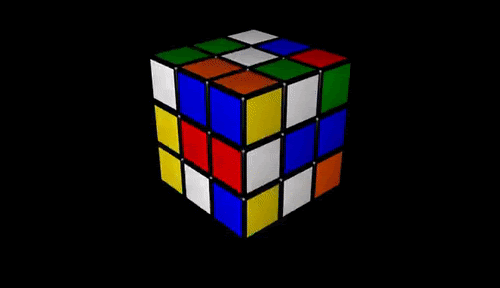Search
•Jeremy Rutman

# Rubik's Cube

Updated: Nov 6, 2020Rubik's cube in action

How many states does a Rubik's cube have? The 'cubelets' or 'cubies' are of three varieties: corner pieces (with three faces), edge pieces (with two faces), and center pieces (with one face). These three types are atomic in the sense that a corner remains a corner, an edge remains an edge, and a center remains a center (even if you break apart the cube and put it back together solved...but we'd never think of it).The patented "Rubik's Cube"

If each of the 8 corners could be put in any of the 8 corner locations there would be 8*7*6*5*4*3*2*1 = 8! possible ways to arrange them. Each of the corners can also be rotated into one of 3 orientations, so there are 3^8 possible orientations for each positional arrangement.

Likewise each of the 12 edges can be put in any of the 12 edge locations there would be 12! possible ways to arrange them. Each of the edges can also be flipped into one of 2 orientations, so there are 2^12 possible orientations for each positional arrangement. So we hit the numberIt turns out that there is not complete freedom in cube arrangements however; just as there is no way to get two opposing centers out of opposition (e.g. the blue center is always opposite the white center on an original cube) , there is no way to do a series of Rubik's moves that rotates one corner and leaves everything else as-is; at the very least two corners will be rotated and not just one. This is built into how the cube operates, as gravity is built into our universe.

Likewise for the edges; you can't flip a single edge while leaving everything else the same. It turns out that we'll have to divide by a factor of 3 for the corners, 2 for the edges, and there's another factor of two in the mix giving:If you've ever tried to solve the cube you may have wondered how many steps are required, if you did everything exactly right; but obviously this depends on how mixed up the cube is. So there must be a 'most mixed up' state which requires the most moves to solve even were God himself solving the cube (by means of the regular rotations and not some supernatural miracle-type move). How many moves are required to solve the 'most mixed state'? That number is 'God's Number' amongst cube-math aficionados and you can actually easily find a lower bound for this number as follows.

We'll take a solved cube and see how many states are possible after N moves. If we hit more than the number of possible states we know we've gone past God's number. There are twelve possible 'atomic moves' to do on a cube, namely a 90 degree and -90 degree rotation for each of the six faces:Rubiks Moves 

Anything else (like a 180 degree rotation) can be composed of those atomic moves.

So after one move we have 12 possible states. From each of those states, 11 lead to new states and one goes back to the old state so we have an inequalityOne can solve that with two lines of python:

```for i in range(20):
print(i+1,' moves ',12*11**i,' states')```

1 moves 12 states

2 moves 132 states

3 moves 1452 states

4 moves 15972 states

5 moves 175692 states

6 moves 1932612 states

7 moves 21258732 states

8 moves 233846052 states

9 moves 2572306572 states

10 moves 28295372292 states

11 moves 311249095212 states

12 moves 3423740047332 states

13 moves 37661140520652 states

14 moves 414272545727172 states

15 moves 4556998002998892 states

16 moves 50126978032987812 states

17 moves 551396758362865932 states

18 moves 6065364341991525252 states

19 moves 66719007761906777772 states --> this one is larger than 4.3*10^19

So God's number is at least 18. There were also results for an upper bound, that is to say that God's number can be at most 52 (proven in 1981). To cut a 29-year story short in which the upper limit dropped and the lower limit rose, in the year 2010 some blokes managed to bridge the gap (between upper and lower limit) and show that God's number is 20. Only a small fraction of states require 20 moves to solve; most actually take 18 moves for God to solve as you can see on the plot below (which shows the number of states requiring a given number N of moves to solve).Algorithms for solving the cube that humans are able to memorize usually involve 50-150 moves and 20-50 different sub-algorithms for different situations.

The states of the cube can be drawn as nodes of a graph and the moves as edges, producing such beauties as the following:rubik's graph of states

This is a 'subgroup of Rubik's mini group'  - for more info on group theory and the cube see .

Interestingly, Adi Shamir gave an early algorithm  on how to solve the cube. Shamir as you may recall is one of the guys behind the RSA assymetric crypto that gives us https (the secure system ensuring that web traffic can't be snooped upon). For current-event points (this being written October 2020, just before US presidential elections) he also gives a secure voting system based on 'homomorphic secret sharing' .

 https://web.mit.edu/sp.268/www/rubik.pdf

 https://miscellaneouscoder.wordpress.com/2014/08/11/an-algorithm-for-simplifying-graphs/